# Diophantine equations

(diff) ← Older revision | Latest revision (diff) | Newer revision → (diff)

Algebraic equations, or systems of algebraic equations with rational coefficients, the solutions of which are sought for in integers or rational numbers. It is usually assumed that the number of unknowns in Diophantine equations is larger than the number of equations; thus, they are also known as indefinite equations. In modern mathematics the concept of a Diophantine equation is also applied to algebraic equations the solutions of which are sought for in the algebraic integers of some algebraic extension of the fieldof rational numbers, of the field of-adic numbers, etc.

The study of Diophantine equations is on the border-line between number theory and algebraic geometry (cf. Diophantine geometry).

Finding solutions of equations in integers is one of the oldest mathematical problems. As early as the beginning of the second millennium B.C. ancient Babylonians succeeded in solving systems of equations with two unknowns. This branch of mathematics flourished to the greatest extent in Ancient Greece. The principal source is Aritmetika by Diophantus (probably the 3rd century A.D.), which contains different types of equations and systems. In this book, Diophantus (hence the name "Diophantine equations" ) anticipated a number of methods for the study of equations of the second and third degrees which were only fully developed in the 19th century . The creation of the theory of rational numbers by the scientists of Ancient Greece led to the study of rational solutions of indefinite equations. This point of view is systematically followed by Diophantus in his book. Even though his work contains solutions of specific Diophantine equations only, there is reason to believe that he was also familiar with a few general methods.

The study of Diophantine equations usually involves major difficulties. Moreover, it is possible to specify, explicitly, polynomials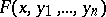with integer coefficients such that no algorithm exists by which it would be possible to tell, for any given, whether the equation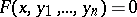is solvable for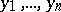(cf. Diophantine equations, solvability problem of). Examples of such polynomials may be explicitly written down; no exhaustive description of their solutions can be given (if the Church thesis is accepted).

The simplest Diophantine equation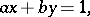whereandare relatively prime integers, has infinitely many solutions (if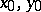form a solution, then the pair of numbersand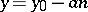, whereis an arbitrary integer, will also be a solution). Another example of a Diophantine equation isPositive integral solutions of this equation represent the lengths of the small sidesand of the hypotenuseof right-angled triangles with integral side lengths; these numbers are known as Pythagorean numbers. All triplets of relatively prime Pythagorean numbers are given by the formulas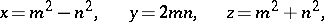whereandare relatively prime integers ().

Diophantus in his Aritmetika deals with the search for rational (not necessarily integral) solutions of special types of Diophantine equations. The general theory of solving of Diophantine equations of the first degree was developed by C.G. Bachet in the 17th century; for more details on this subject see Linear equation. P. Fermat, J. Wallis, L. Euler, J.L. Lagrange, and C.F. Gauss in the early 19th century mainly studied Diophantine equations of the form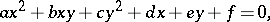where,,,,, andare integers, i.e. general inhomogeneous equations of the second degree with two unknowns. Lagrange used continued fractions in his study of general inhomogeneous Diophantine equations of the second degree with two unknowns. Gauss developed the general theory of quadratic forms, which is the basis of solving certain types of Diophantine equations.

In studies on Diophantine equations of degrees higher than two significant success was attained only in the 20th century. It was established by A. Thue that the Diophantine equation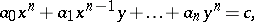where,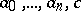are integers, and the polynomial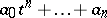is irreducible in the field of rational numbers, cannot have an infinite number of integer solutions. However, Thue's method fails to yield either a bound on the solutions or on their number. A. Baker obtained effective theorems giving bounds on solutions of certain equations of this kind. B.N. Delone proposed another method of investigation, which is applicable to a narrower class of Diophantine equations, but which yields a bound for the number of solutions. In particular, Diophantine equations of the form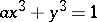are fully solvable by this method.

The theory of Diophantine equations has many directions. Thus, a well-known problem in this theory is Fermat's problem — the hypothesis according to which there are no non-trivial solutions of the Diophantine equation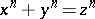(1)

if. The study of integer solutions of equation (1) is a natural generalization of the problem of Pythagorean triplets. Euler obtained a positive solution of Fermat's problem for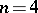. Owing to this result, Fermat's problem is reduced to the proof of the absence of non-zero integer solutions of equation (1) ifis an odd prime. At the time of writing (1988) the study concerned with solving (1) has not been completed. The difficulties involved in solving it are due to the fact that prime factorization in the ring of algebraic integers is not unique. The theory of divisors in rings of algebraic integers makes it possible to confirm the validity of Fermat's theorem for many classes of prime exponents.

The arithmetic of rings of algebraic integers is also utilized in many other problems in Diophantine equations. For instance, such methods were applied in a detailed solution of an equation of the form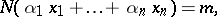(2)

whereis the norm of the algebraic number, and integral rational numberswhich satisfy equation (2) are to be found. Equations of this class include, in particular, the Pell equation. Depending on the values ofwhich appear in (2), these equations are subdivided into two types. The first type — the so-called complete forms — comprises equations in which among the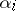there arelinearly independent numbers over the field of rational numbers, where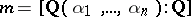is the degree of the algebraic number field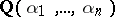over. Incomplete forms are those in which the maximum number of linearly independent numbersis less than. The case of complete forms is simpler and its study has now, in principle, been completed. It is possible, for example, to describe all solutions of any complete form .

The second type — the incomplete forms — is more complicated, and the development of its theory is still (1988) far from being completed. Such equations are studied with the aid of Diophantine approximations. They include the equationwhereis an irreducible homogeneous polynomial of degree. This equation may be written as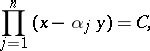(3)

where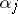are all the roots of the polynomial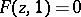. The existence of an infinite sequence of integral solutions of equation (3) would lead to relationships of the form(4)

for some. Without loss of generality, one may assume that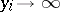. Accordingly, if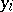is sufficiently large, inequality (4) will be in contradiction with the Thue–Siegel–Roth theorem, from which follows that the equation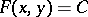, whereis an irreducible form of degree three or higher, cannot have an infinite number of solutions.

Equations such as (2) constitute a fairly narrow class among all Diophantine equations. For instance, their simple appearance notwithstanding, the equations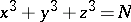(5)

and(6)

are not in this class. The study of the solutions of equation (6) is a fairly thoroughly investigated branch of Diophantine equations — the representation of numbers by quadratic forms. The Lagrange theorem states that (6) is solvable for all natural. Any natural number not representable in the form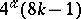, whereandare non-negative integers, can be represented as a sum of three squares (Gauss' theorem). Criteria are known for the existence of rational or integral solutions of equations of the form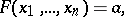where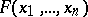is a quadratic form with integer coefficients. Thus, according to Minkowski–Hasse theorem, the equationwhereandare rational, has a rational solution if and only if it is solvable in real numbers and in-adic numbers for each prime number.

The representation of numbers by arbitrary forms of the third degree or higher has been studied to a lesser extent, because of inherent difficulties. One of the principal methods of study in the representation of numbers by forms of higher degree is the method of trigonometric sums (cf. Trigonometric sums, method of). In this method the number of solutions of the equation is explicitly written out in terms of a Fourier integral, after which the circle method is employed to express the number of solutions of the equation in terms of the number of solutions of the corresponding congruences. The method of trigonometric sums depends less than do other methods on the algebraic peculiarities of the equation.

There exists a large number of specific Diophantine equations which are solvable by elementary methods .

How to Cite This Entry:
Diophantine equations. Encyclopedia of Mathematics. URL: http://encyclopediaofmath.org/index.php?title=Diophantine_equations&oldid=13828
This article was adapted from an original article by S.M. Voronin (originator), which appeared in Encyclopedia of Mathematics - ISBN 1402006098. See original article